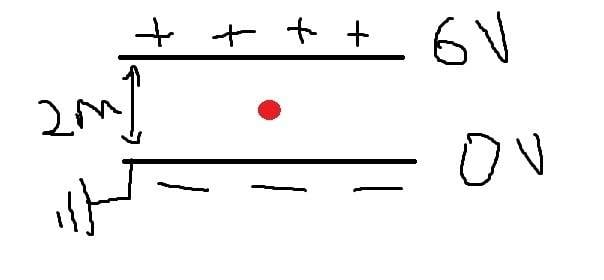# Electric Field and Force between two plates [homework question]

## Homework Statement

*Is the a difference when calculating the electric field , or a force exerted on a charge between two plates , if the two plates were :
a) both plates are charged ( connected to a power source) ,
b) both plates are charged ( connected to a power source), but the negative plate is earthed , i.e 0 V.

*Do we consider the sign of the charge when calculating the electric field ?

E=F/Q or F=EQ
E=V/d
F=e V/d

## The Attempt at a Solution

BiGyElLoWhAt
Gold Member
That blank attempt at the solution is somewhat bothersome. What's the voltage across the gap between the plates in either case?

That blank attempt at the solution is somewhat bothersome. What's the voltage across the gap between the plates in either case?
Im just asking a general question, not a specific problem ,, take any voltage you like

BiGyElLoWhAt
Gold Member
Well I should really treat this like a homework problem. So the question becomes, in case a (nongrounded) lets say it's -3 and +3V. Is the second case 0 and +3 or 0 and +6?

Well I should really treat this like a homework problem. So the question becomes, in case a (nongrounded) lets say it's -3 and +3V. Is the second case 0 and +3 or 0 and +6?
exactly
i uploaded a pic as an example for the second case , how do find the the electric field strength , and force on the electron if it was ,lets say, at mid point between the plates ?BiGyElLoWhAt
Gold Member
Np, this is physics forum not art forum. If you look at your relevent equations, and then look at your picture, you have a voltage and a distance; you're looking for the electric field. You have the equation you need, with one (reasonable) assumption. First off, 2m is pretty large for a gap in capacitors, but it doesn't really matter, as long as we can assume that the length of the plates are long with respect to the gap, the field will be approximately uniform throughout the region between the plates. Then it's a matter of applying coloumbs law once you find the field. This will give you the force on the electron.

With our assumption, it doesn't matter if the electron was mid point, quarter way or pi/32 of the way through, the force would be (for all intensive purposes) the same.

Np, this is physics forum not art forum. If you look at your relevent equations, and then look at your picture, you have a voltage and a distance; you're looking for the electric field. You have the equation you need, with one (reasonable) assumption. First off, 2m is pretty large for a gap in capacitors, but it doesn't really matter, as long as we can assume that the length of the plates are long with respect to the gap, the field will be approximately uniform throughout the region between the plates. Then it's a matter of applying coloumbs law once you find the field. This will give you the force on the electron.

With our assumption, it doesn't matter if the electron was mid point, quarter way or pi/32 of the way through, the force would be (for all intensive purposes) the same.

oh lol i meant 2 cm ,nevermind ,,
so the position doesnt matter i see , what about if the electron was moving , for example from the negative plate to mid point ,, is it the same case ?

BiGyElLoWhAt
Gold Member
Essentially, yes. If you want to find out for sure. Assume uniform charge density on either plate, the integrate the field contribution from each dL along the length of the plate for some arbitrary point (x,y) (you only need to do this in 2d to meet your picture). If you do that for both plates, then apply superposition and sum the contributions from both plates for all points (x,y) you will see that all points are approximately the same, especially far from the edges.

Essentially, yes. If you want to find out for sure. Assume uniform charge density on either plate, the integrate the field contribution from each dL along the length of the plate for some arbitrary point (x,y) (you only need to do this in 2d to meet your picture). If you do that for both plates, then apply superposition and sum the contributions from both plates for all points (x,y) you will see that all points are approximately the same, especially far from the edges.
that explains ,thanks
when I learned about equipotentials , i got confused ..
so even if one plate was at 0V , the electric field strength stays constant at all points - (just to make sure)

BiGyElLoWhAt
Gold Member
Yea, zero potential doesn't really mean anything. It's kind of like me saying that a block sitting on a table has 0 GPE or me saying that it has GPE mg(height of the table from the floor). Very analagous.

Yea, zero potential doesn't really mean anything. It's kind of like me saying that a block sitting on a table has 0 GPE or me saying that it has GPE mg(height of the table from the floor). Very analagous.
alright ,, thanks a lot for helping :)